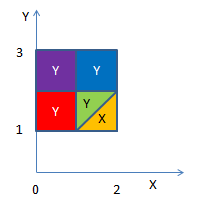#### X,Y 独立并且，X~U(0,2)以及Y∼U(1,3)。求max(X,Y) 的期望。

统计/机器学习 概率分布    浏览次数：1467        分享

0Zero1   2019-09-05 09:13

##### 2个回答
4import numpy as np
n=100000
x = np.random.uniform(0, 2,n)
y = np.random.uniform(1, 3,n)
z=np.maximum(x,y)
print(np.mean(z))

2.0405704490035905

SofaSofa数据科学社区DS面试题库 DS面经Zealing   2019-09-06 08:45

2

import numpy as np
x = np.random.uniform(0, 2, 1000000)
y = np.random.uniform(1, 3, 1000000)
print(np.mean(np.max([x, y], axis=0)))

SofaSofa数据科学社区DS面试题库 DS面经houherui   2019-09-06 05:08相关主题